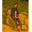Related Tags

c++
arrays

# How to dynamically allocate, initialize, and delete arrays in C++Arham Qayyum

### Arrays in C++

Arrays are used to store more than one value in a single variable, instead of making different variables for each value.

### Example

In the example below, an array cars is declared on the stack of fixed size 3. It is then initialized with the values: "City", "Corolla", and "Vitz", respectively.

#include <iostream>
#include <string>

using namespace std;

int main() {
string cars = {"City" , "Corolla" , "Vitz"};

for (int i = 0; i < 3; i++) {
cout << "Car " << i << ": " << cars[i] << endl;
}

return 0;
}

### Dynamically allocated arrays

Heap allows us to allocate memory at runtime. Therefore, dynamically allocated arrays can have variable lengths.

### Syntax

data_type * variable_name = new data_type[size];


The above syntax declares a variable on the stack, variable_name. This is a pointer variable since it has a * after the data_type. The new keyword allocates memory on the heap for the specified data_type and size.

### Example

In the example below, the size of the array is taken as input from the user and stored in the length variable. Then, on line 9, a pointer to an int array is declared as arr and is assigned an int array of the given length. The first for loop stores the multiplies of ten and the second for loop prints the contents of the array.

#include <iostream>
using namespace std;

int main() {
int length;

cin >> length;

int * arr = new int[length];

for (int i = 0; i < length; i++) {
arr[i] = (i + 1) * 10;
}

for (int i = 0; i < length; i++) {
cout << arr[i] << " " << endl;
}

return 0;
}

Enter the input below

### Dynamically delete arrays

To delete a dynamic array, the delete or delete[] operator is used. It deallocates the memory from heap. The delete[] keyword deletes the array pointed by the given pointer.

Therefore, to delete a dynamically allocated array, we use the delete[] operator.

Note: If only a single element is declared on the heap, then the delete operator is used instead (without the square brackets []).

### Example

The example below is a modified version of the previous example that also deallocates the memory of the arr at the end of the program.

#include <iostream>
using namespace std;

int main() {
int length;

cin >> length;

int * arr = new int[length];

for (int i = 0; i < length; i++) {
arr[i] = (i + 1) * 10;
}

for (int i = 0; i < length; i++) {
cout << arr[i] << " " << endl;
}

delete[] arr;

return 0;
}

Enter the input below

RELATED TAGS

c++
arrays

CONTRIBUTORArham Qayyum
RELATED COURSES

View all Courses

Keep Exploring

Learn in-demand tech skills in half the time